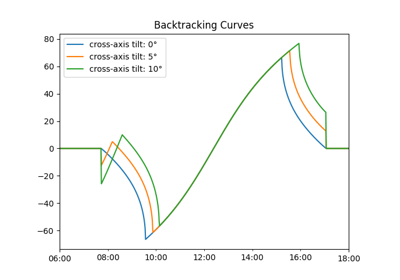# pvlib.tracking.calc_cross_axis_tilt#

pvlib.tracking.calc_cross_axis_tilt(slope_azimuth, slope_tilt, axis_azimuth, axis_tilt)[source]#

Calculate the angle, relative to horizontal, of the line formed by the intersection between the slope containing the tracker axes and a plane perpendicular to the tracker axes.

Use the cross-axis tilt to avoid row-to-row shade when backtracking on a slope not parallel with the axis azimuth. Cross-axis tilt should be specified using a right-handed convention. For example, trackers with axis azimuth of 180 degrees (heading south) will have a negative cross-axis tilt if the tracker axes plane slopes down to the east and positive cross-axis tilt if the tracker axes plane slopes down to the west.

Parameters
• slope_azimuth (float) – direction of the normal to the slope containing the tracker axes, when projected on the horizontal [degrees]

• slope_tilt (float) – angle of the slope containing the tracker axes, relative to horizontal [degrees]

• axis_azimuth (float) – direction of tracker axes projected on the horizontal [degrees]

• axis_tilt (float) – tilt of trackers relative to horizontal. `axis_tilt` must be >= 0 and <= 90. [degree]

Returns

cross_axis_tilt (float) – angle, relative to horizontal, of the line formed by the intersection between the slope containing the tracker axes and a plane perpendicular to the tracker axes [degrees]

Notes

See 1 for derivation of equations.

References

1

Kevin Anderson and Mark Mikofski, “Slope-Aware Backtracking for Single-Axis Trackers”, Technical Report NREL/TP-5K00-76626, July 2020. https://www.nrel.gov/docs/fy20osti/76626.pdf

## Examples using `pvlib.tracking.calc_cross_axis_tilt`#Backtracking on sloped terrain

Backtracking on sloped terrain